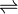# Snapquiz - Percentage Yield

This is the Snapquiz on Percentage Yield. Click here or use the embedded video if you haven't watched the lesson yet.

Question 1. What does this symboltell us about a reaction?

It's unstable.
It's irreversible.
It's reversible.
It's impossible.

Question 2. What is the maximum possible yield called?

Total yield.
Maximum yield.
Actual yield.
Theoretical yield.

Question 3. Which number goes on the top of your fraction when you're calculating a percentage on a foundation level GCSE Science paper?

100%.
The large number.
The small number.
The denominator.

Question 4. What is three kilograms as a percentage of five kilograms?

30%.
50%.
60%.
40%.

Question 5. Which compound is manufactured in the Haber Process?

Calcium Carbonate.
Ethanol.
Ammonia.
Hydrochloric Acid.